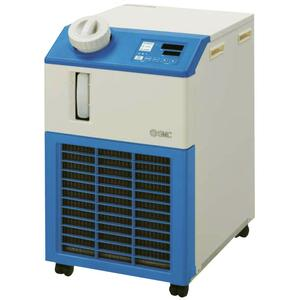# HRS, General Use Compact Chiller, 230VAC• Cooling capacities: 1.3 kW, 1.9 kW, 2.4 kW, 3.2 kW, 4.2 kW, 5.1 kW, 5.9 kW (at 60Hz)
• Temperature range setting: 5 to 40°C
• Temperature stability: ±0.1°C
• Power supply requirement: single phase 200 to 230 VAC, 50/60Hz
• Standards: CE, UL, RoHS
• Circulating fluid: Tap water or 15% ethylene glycol, 30% Dowcal™100, ControXic 1642, Hexid A4, Coolflow IGE, NALCO®CCL105
• SMC recommends Bypass Piping Set HRS-BP001 for Models HRS-012, HRS018, HRS024, HRS030
• SMC recommends Bypass Piping Set HRS-BP004 for Models HRS-040, HRS050, HRS060
Configuration OptionsCopy Configuration Number

## Product Options

Select Product Options
 Select values from the drop down lists or make appropriate selections to configure the part number. Cooling Capacity -- Pick an Option -- 012 (Cooling Capacity: 1100/1300W, 50/60 Hz) 018 (Cooling Capacity: 1700/1900W, 50/60 Hz) 024 (Cooling Capacity: 2100/2400W, 50/60 Hz) 030 (Cooling Capacity: 2600/3200W, 50/60 Hz) 040 (Cooling Capacity: 3800W/4200W, 50/60 Hz) 050 (Cooling Capacity: 4700/5100W, 50/60 Hz) 060 (Cooling Capacity: 4900/5900, 50/60 Hz)Cooling Method -- Pick an Option -- A (Air-cooled Refrigeration) W (Water-cooled Refrigeration)Thread -- Pick an Option -- Rc N (NPT w/PT-NPT Conversion Fitting Set) F (G w/PT-G Conversion Fitting Set)Option -- Pick an Option -- None B (Earth Leakage Breaker) G (High Temp Environment Specs) J (Automatic Water Supply Function) M [Applicable to DI Water (Deionized Water) Piping] T (High-lift Pump) W (Fixed SI Unit) BG (B + G) BJ (B + J) BM (B + M) BT (B + T) BW (B + W) GJ (G + J) GM (G + M) GT (G + T) GW (G + W) JM (J + M) JT (J + T) JW (J + W) MT (M + T) MW (M + W) TW (T + W) BGJ (B + G + J) BGM (B + G + M) BGT (B + G + T) BGW (B + G + W) BJM (B + J + M) BJT (B + J + T) BJW (B + J + W) BMT (B + M + T) BMW (B + M + W) BTW (B + T + W) GJM (G + J + M) GJT (G + J + T) GJW (G + J + W) GMT (G + M + T) GMW (G + M + W) GTW (G + T + W) JMT (J + M + T) JMW (J + M + W) JTW (J + T + W) MTW (M + T + W) BGJM (B + G + J + M) BGJT (B + G + J + T) BGJW (B + G + J + W) BGMT (B + G + M + T) BGMW (B + G + M + W) BGTW (B + G + T + W) BJMT (B + J + M + T) BJMW (B + J + M + W) BJTW (B + J + T + W) BMTW (B + M + T + W) GJMT (G + J + M + T) GJMW (G + J + M + W) GJTW (G + J + T + W) GMTW (G + M + T + W) JMTW (J + M + T + W) BGJMT (B + G + J + M + T) BGJMW (B + G + J + M + W) BGJTW (B + G + J + T + W) BGMTW (B + G + M + T + W) BJMTW (B + J + M + T + W) GJMTW (G + J + M + T + W) BGJMTW (B + G + J + M + T + W)Options in: Gray are not compatible with other selected option value. Red conflict with another selected option value. Amber are not recommended.

Model does not exist, please email cad@famulsl.com彩票大赢家 with the complete part number, CAD file format and version required.